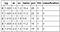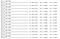# Chronic Kidney Disease Prediction Using Python & Machine Learning

## A Python Program to Detect and Classify Chronic Kidney Disease

In this article I will show you how to create your own python program to predict and classify patience as having chronic kidney disease (ckd) or not using artificial neural networks.

Chronic kidney disease, also called chronic kidney failure, describes the gradual loss of kidney function. Your kidneys filter wastes and excess fluids from your blood, which are then excreted in your urine. When chronic kidney disease reaches an advanced stage, dangerous levels of fluid, electrolytes and wastes can build up in your body. -

In the early stages of chronic kidney disease, you may have few signs or symptoms. Chronic kidney disease may not become apparent until your kidney function is significantly impaired. -

Treatment for chronic kidney disease focuses on slowing the progression of the kidney damage, usually by controlling the underlying cause. Chronic kidney disease can progress to end-stage kidney failure, which is fatal without artificial filtering (dialysis) or a kidney transplant. -

`Data Set Column Information:age	-	age	bp	-	blood pressure sg	-	specific gravity al	-       albumin su	-	sugar rbc	-	red blood cells pc	-	pus cell pcc	-	pus cell clumps ba	-	bacteria bgr	-	blood glucose random bu	-	blood urea sc	-	serum creatinine sod	-	sodium pot	-	potassium hemo	-	hemoglobin pcv	-	packed cell volume wc	-	white blood cell count rc	-	red blood cell count htn	-	hypertension dm	-	diabetes mellitus cad	-	coronary artery disease appet	-	appetite pe	-	pedal edema ane	-	anemia class	-	classification`

If you prefer not to read this article and would like a video representation of it, you can check out the below. It goes through everything in this article with a little more detail, and will help make it easy for you to start programming your own Machine Learning model even if you don’t have the programming language Python installed on your computer. Or you can use both as supplementary materials for learning about Machine Learning !

# Programming:

The first thing that I like to do before writing a single line of code is to put in a description in comments of what the code does. This way I can look back on my code and know exactly what it does.

`#Description: Classify patients as having chronic kidney disease #             or not using Artificial Neural Networks`

Import the libraries

`#Import Librariesimport globfrom keras.models import Sequential, load_modelimport numpy as npimport pandas as pdimport keras as kfrom keras.layers import Densefrom sklearn.model_selection import train_test_splitfrom sklearn.preprocessing import LabelEncoder, MinMaxScalerimport matplotlib.pyplot as plt`

`    #load the data     from google.colab import files #Only use for Google Colab    uploaded = files.upload()      #Only use for Google Colab    df = pd.read_csv("kidney_disease.csv")        #Print the first 5 rows    df.head()`

Get the number of rows and columns in the data set. Remember each row represents a patient and each column is a data point on that patient.

`#Get the shape of the data (the number of rows & columns)df.shape`

# Data Manipulation: Clean The Data

Now we will transform the data. By getting rid of missing data and removing some columns. First we will create a list of column names that we want to keep or retain.

Next we drop or remove all columns except for the columns that we want to retain.

Finally we drop or remove the rows that have missing values from the data set.

`#Create a list of columns to retaincolumns_to_retain = ["sg", "al", "sc", "hemo",                         "pcv", "wbcc", "rbcc", "htn", "classification"]#columns_to_retain = df.columns, Drop the columns that are not in columns_to_retaindf = df.drop([col for col in df.columns if not col in columns_to_retain], axis=1)    # Drop the rows with na or missing valuesdf = df.dropna(axis=0)`

Let’s loop through all of the columns and find the columns that do not contain number values. For those columns we will transform the values into numeric data.

`#Transform non-numeric columns into numerical columnsfor column in df.columns:        if df[column].dtype == np.number:            continue        df[column] = LabelEncoder().fit_transform(df[column])`

We will print the first 5 rows of the new data set.

`df.head()`Fig 2 : Sample of the first 5 rows of new data set

# Data Manipulation: Split & Scale The Data

Let’s split the data set into a independent data set that we will call `X` which is the feature data set and a dependent data set that we will call `y` which is the target data set.

`#Split the dataX = df.drop(["classification"], axis=1)y = df["classification"]`

Next we will scale the feature data set to be values between 0 and 1 inclusively.

`#Feature Scalingx_scaler = MinMaxScaler()x_scaler.fit(X)column_names = X.columnsX[column_names] = x_scaler.transform(X)`

Once we are done with all of that, we will split the data sets into 80% training (`X_train` and `y_train`) and 20% testing (`X_test` and `y_test`) data sets, and shuffle the data before training.

`#Split the data into 80% training and 20% testing X_train,  X_test, y_train, y_test = train_test_split(        X, y, test_size= 0.2, shuffle=True)`

# Build The Model (Artificial Neural Network):

We are ready to build the model also known as the Artificial Neural Network !
First we must create the models architecture, then we will add 2 layers, the first layer with 256 neurons and the ‘ReLu activation function with a normal distribution initializer for the weights. Since that layer is the first layer we must also specify the number of features/columns in the data set `len(X.columns)`.

The second layer which happens to be the last layer as well, will have 1 neuron and use the ‘hard_sigmoid’ activation function.

`#Build The modelmodel = Sequential()model.add(Dense(256, input_dim=len(X.columns),              kernel_initializer=k.initializers.random_normal(seed=13), activation="relu"))model.add(Dense(1, activation="hard_sigmoid"))`

Compile the model, and give it the loss function called ‘binary_crossentropy’ which is a loss function used for binary classification, it measures how well the model did on training and then tries to improve on it using the optimizer.

The optimizer that we will give it is called the ‘adam’ optimizer. We also want to see how well the model does, so we will get some metrics on the models accuracy.

`#Compile the modelmodel.compile(loss='binary_crossentropy',                   optimizer='adam', metrics=['accuracy'])`

Train the model using the training data sets (`X_train` and `y_train`). Give it 2000 epcochs and a batch size equal to the number of patients/rows in the data set.

Batch: Total number of training examples present in a single batch

Epoch:The number of iterations when an ENTIRE dataset is passed forward and backward through the neural network only ONCE.

Fit: Another word for train

`#Train the modelhistory = model.fit(X_train, y_train,                     epochs=2000,                     batch_size=X_train.shape) `Fig 3: A sample of the training with the models accuracy = 99.56% and loss= .0087

Now that we are done creating our model. Let’s save it.

`#Save the modelmodel.save("ckd.model")`

Visualize how well the model did on the training data set by plotting the loss and accuracy of the model.

`#Visualize the models accuracy and lossplt.plot(history.history["acc"])plt.plot(history.history["loss"])plt.title("model accuracy & loss")plt.ylabel("accuracy and loss")plt.xlabel("epoch")plt.legend(['acc', 'loss'], loc='lower right')plt.show()`

Get the training and test data shape

`print("---------------------------------------------------------")print("Shape of training data: ", X_train.shape)print("Shape of test data    : ", X_test.shape )print("---------------------------------------------------------")`

Loop through any and all saved models. Then get each models accuracy, loss, prediction and original values on the test data.

`for model_file in glob.glob("*.model"):  print("Model file: ", model_file)  model = load_model(model_file)  pred = model.predict(X_test)  pred = [1 if y>=0.5 else 0 for y in pred] #Threshold, transforming probabilities to either 0 or 1 depending if the probability is below or above 0.5  scores = model.evaluate(X_test, y_test)  print()  print("Original  : {0}".format(", ".join([str(x) for x in y_test])))  print()  print("Predicted : {0}".format(", ".join([str(x) for x in pred])))  print()   print("Scores    : loss = ", scores, " acc = ", scores)  print("---------------------------------------------------------")  print()`

# Conclusion and Resources

That is it, you are done creating your program to predict if a patient has chronic kidney disease or not!

Again, if you want, you can watch and listen to me explain all of the code in my .

If you are interested in reading more about machine learning to immediately get started with problems and examples, I recommend you read

It is a great book for helping beginners learn to write machine-learning programs and understanding machine-learning concepts.

# Other resources

1. Dua, D. and Graff, C. (2019). UCI Machine Learning Repository [http://archive.ics.uci.edu/ml]. Irvine, CA: University of California, School of Information and Computer Science.